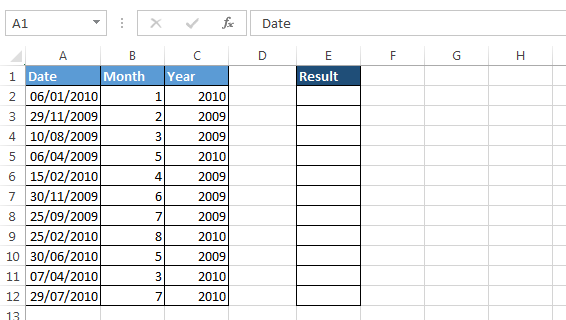# Count the number of times dates in certain month appears in range in Microsoft Excel

In this article, you will learn how to count the number of times dates in certain month appear in range in Microsoft Excel. To solve this problem we use SUM function along with MONTH and YEAR function.

In this problem:-

• SUM function will help to return the count on the basis of criteria.
• MONTH function will help to provide the month for matching.
• YEAR function will help to provide the year for matching.

Let’s understand with example:-

We have a range of date and in other ranges we have criteria list of month and Year.• Enter the formula in cell E2
• =SUM((MONTH(\$A\$2:\$A\$12)=B2)*(YEAR(\$A\$2:\$A\$12)=C2)*1)
• Press the key Ctrl+Shift+Enter
• The function will return 1
• Copy the same formula in the range.
• How to count the numbers through SUM formula
• How to count on the basis of Month and Year (multiple criteria)